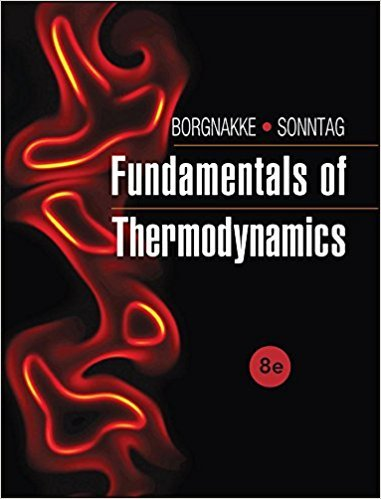×
Get Full Access to Fundamentals Of Thermodynamcs - 8 Edition - Chapter 2 - Problem 88hp
Get Full Access to Fundamentals Of Thermodynamcs - 8 Edition - Chapter 2 - Problem 88hp

×

# What is the percent error in specific volume if the idealISBN: 9781118131992 58

## Solution for problem 88HP Chapter 2

Fundamentals of Thermodynamcs | 8th Edition

• Textbook Solutions
• 2901 Step-by-step solutions solved by professors and subject experts
• Get 24/7 help from StudySoup virtual teaching assistantsFundamentals of Thermodynamcs | 8th Edition

4 5 1 408 Reviews
21
4
Problem 88HP

Problem 88HP

What is the percent error in specific volume if the ideal gas model is used to represent the behavior of superheated ammonia at 40°C and 500 kPa? What if the generalized compressibility chart, Fig. D.1, is used instead?

FIGURE D.1 Lee–Kesler simple fluid compressibility factor.Step-by-Step Solution:

Solution 88HP

Step 1 of 5

The objective here is to determine the error percentage in two cases:

1.  If the ideal gas model is used to represent the behavior of superheated ammonia under the given conditions.
2. Using the Compressibility chart.

Part (a)

Step 2 of 5

Step 3 of 5

##### ISBN: 9781118131992

Unlock Textbook Solution